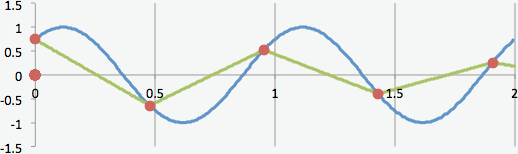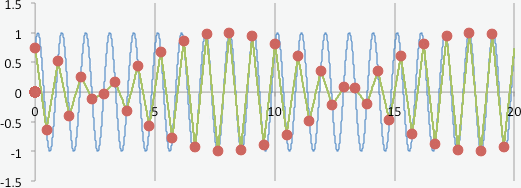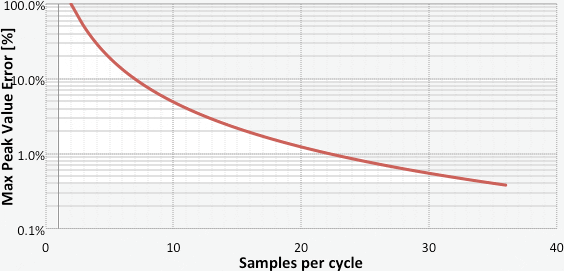Home/Resources/Ask The Experts/Shock measurements: An appropriate sampling rate

# Shock measurements: An appropriate sampling rate

### Question

I am carrying out shock acceleration measurements. My goal is to record shock transients in a frequency bandwidth up to 4 kHz. The parameters of signal recording are:

• Sampling rate: 10 kHz
• Anti-aliasing filter: 4kHz (-3dB)

The lack of repetitive results raises my concerns. Amplitude readouts differ significantly between the measurements, so their repeatability is far below the expectations. I have double checked continuity of the connection between sensor and my Data Acquisition System (DAQ). I am sure that the measurement chain is ok and it remains stable during the tests. Can you help identify the source of my problems?

The source of your problem is probably due to an insufficient sampling rate. The current sampling rate does not let you carry out precise observations of the transient phenomenon. If the current- sampling rate is inappropriate, then what is the correct sampling rate? How many samples per period shall be applied to obtain reliable results?

In case of periodic, stationary signals  the sampling rate shall be at least two times the highest frequency of our interest. It may seem to be quite surprising, but it is possible that the continuous time signal can be reconstructed with only e.g. 2.1 samples per period only [1, 2]. The process of reconstruction is called upsampling and it requires application of some interpolation methods, which is beyond the scope of this paper. What is obvious is that the raw digital data points collected with 2.1 samples per cycle do not represent the original signal well .

Below example signal plots are showing two (fig. 1) and twenty (fig. 2) periods of the same continuous signal sampled with 2.1 samples per period sampling rate.Figure 1Figure 2

It is clearly visible that the correct amplitude indication depends on phase match between sampling and sampled signal. In the case of periodic, stationary signals  those raw digital data points are the information sufficient to perform RMS calculation, digital Fourier transform and all spectral analysis related to it, in spite of the fact that signal representation may not be always precise. Therefore the number of samples per period of highest frequency signal components is rarely one of the major concerns of engineers carrying out signal spectral analysis

In case of transient signals which are not periodic or stationary, the results of RMS or peak value estimation, based on digital data points that are an imprecise representation of original time signal, may contain a significant error. The phase relations between sampling rate and high frequency will be random, what will result are random errors in our measurements, even if the acceleration transient will be repeated with exactly the same parameters.

Let's take a look at the above plot showing twenty periods of periodic signal sampled with a sampling rate of 2.1 samples per period. Let's also assume that our transient signal will contain 3 sine periods only. If the phase match between the sampling and high signal component will be similar to the one we can see between periods 2 and 4, the presence of this signal component may not be even detected.

As the information about all signal components shall be error free, in the case of transient signals the sampling rate must be much higher than two times the sampling rate. Therefore to be sure that the possible amplitude readout error will be limited to <5%, the applied sampling rate shall be 10 times higher than the highest frequency of our interest. Limiting the maximum error down to <1% will impose the sampling rate 23 times higher than the highest frequency of our interest.

The below formula and chart show maximum signal peak value error in the function of samples per period .Figure 3

where N is the number of samples per cycle.Figure 4

The attached excel file allows one to calculate the maximum amplitude measurement error as a function of the number of samples per period. It calculates the maximum possible amplitude error from above formula and also shows and calculates the error for different random signal and sampling rate phase match.

Disclaimer:

Author of the article would like to notice that article's content concerns the theory of digital signal processing only. The point of the article is to increase the level of awareness concerning the errors that may occur at the stage of time signal transformation to its digital form and information loses related to it. In practice measurement error may also depend on number of other factors not related to analogue to digital signal transformation and not mentioned in this article. Their possible appearance and influence also has to be taken into the account.

References:

1. http://en.wikipedia.org/wiki/Nyquist%E2%80%93Shannon_sampling_theorem
2. Allan G. Piersol, Thomas L. Paez; Haris' Shock and Vibration Handbook, chapter 13
"Shock and vibration data acquisition"
3. Allan G. Piersol, Thomas L. Paez; Haris' Shock and Vibration Handbook, chapter 19 "Vibration data analysis"